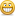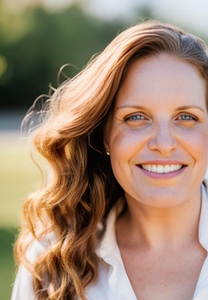# A Solution to rounding in Storyline – without using Javascript

Jun 06, 2013

Normal 0 false false falseoNotPromoteQF /> EN-US X-NONE X-NONE

I found a way to round numbers in Storyline without using Javascript.  The challenge was to show whole percentage numbers from calculated decimal numbers (i.e. 33% for 1/3 and 67% for 2/3).  Searching through the forums, all I could find was Javascript solutions and suggestions to submit a feature request.  Not happy with either of these answers, I continued to play around.

I found that Storyline only holds 7 digits past the decimal place, and the 7th digit is rounded.  So to get a rounded decimal (percentage) as a whole number, divide by 100,000, and then multiply by 10,000,000.

For example:

2 / 3 = 0.6666667  (rounded at the 7th position by Storyline)

0.6666667 / 100,000 = .000006666667 (which is rounded to 0.0000067)

0.0000067 * 10,000,000 = 67

Now we have our rounded 67% from 2/3

You should be able to apply this to any number you want to round.  Divide and then multiply by a multiple of 10 that is large enough to push the number you want to round to the 7th position past the decimal (i.e. 13,567 / 10,000,000,000 * 10,000,000,000 = 14,000)

###### 96 RepliesI'm pretty new to Storyline - only one course produced so far but loving it - and I have been asked by my client if I can get the final score to always be a whole number. I used the trick above to create the variable 'RoundedScore' and it works great, but I don't know how I then get that variable to be the score passed back to the LMS when my 'submit score' button is clicked.

Is this possible?Let me start by saying math isn't my strong suit but I can't seem to get this to work properly with the standard variable Results.ScorePercent. For example my score is 42.85% as reported by the Results.ScorePercent variable. I created a new variable called NonRoundScore as S. Yu suggested and set it to equal Results.ScorePercent. I then set triggers to divide it by 100,000 and then multiply it by 10,000,000. But the result of my NonRoundScore variable = 4285%. What I want it to show is 43%. I'm guessing that it is the numbers that I'm multiplying and dividing by but I can't seem to discover what the right combination is to get me to my magic 43%.

Anyone out there who can lend a hand...?Divide by 10.000,000 and then multiply by the same.

For those who want the math logic behind this answer,

42.85 / 10,000,000 = 0.0000043 in articulate (dividing by 10,000,000 moves the decimal place 7 places to the left and the 7th decimal position gets rounded)

0.0000043 * 10,000,000 = 43.0 (multiplying by 10,000,000 moves the decimal 7 places to the right resulting in a whole number)Just a quick note to say thank and report success using this technique!! I wanted a rounded percentage, so divided by 10000000 then multiplied by the same number did the job.

Craig Bellingham said:

I'm pretty new to Storyline - only one course produced so far but loving it - and I have been asked by my client if I can get the final score to always be a whole number. I used the trick above to create the variable 'RoundedScore' and it works great, but I don't know how I then get that variable to be the score passed back to the LMS when my 'submit score' button is clicked.

Is this possible?

Not sure of how to go about this, but I would suggest storing the actual score, and then customising the score display web page to do the number rounding job – doing this means you are not storing bogus data in your LMS database.I ran into a similar issue and I didn't want to spend the time to make this edit since I only had a 3 question quiz so I found a really good alternative!

All I did was change the question scores from the defaulted 10pts (2 to 33pts and 1 to 34pts) and then changed the results display from %Results.ScorePercent% to %Results.ScorePoints%.  That resulted in making the results rounded in less than a minute!Jennifer Barnett said:

I ran into a similar issue and I didn't want to spend the time to make this edit since I only had a 3 question quiz so I found a really good alternative!

All I did was change the question scores from the defaulted 10pts (2 to 33pts and 1 to 34pts) and then changed the results display from %Results.ScorePercent% to %Results.ScorePoints%.  That resulted in making the results rounded in less than a minute!

this will only work if your points add up yo 100, because you are no longer showing a percentage, points have to be integersThanks for the tip. I needed to have rounded off percentages for my project.

This really should be an option that can be set in the quiz options.

I posted a feature request.

I am including a zipped up sample of the Articulate Storyline file here for others to see how this was set up. There is also a "Instructions" PDF file included with some screenshots of variables setup. My quiz here uses a number of question banks so the questions are randomized.

To sum up what happens is per previous instructions by Terri and others I have created a few triggers that divide and then multiply the quiz results Percentage score by 10000000. It does this to push the score percentage past the Seven digits limit decimal place.I'll try this not for quizzing but simply wanting to display whole % such as % of ee's in one location or another - quizzing must already be displaying %s not in decimal form, since, when working with variables simply on a silde or layer, you must divide by 100,000 & multiply by 10,000,000 to get the correct result.

Don't suppose there's a solution to formatting with commas? for ex: I want the number value (can't be text) of 31000 to display 31,000.Can anyone help me find a fix to my problem.

I have been happily using the rounding up solution posted above (great fix BTW) but I've run into an issue.

I have a screen that calculates stuff.  The main calculation is taking a number and dividing it by 30%.  This solution has worked a treat until we entered the value 30.

30% of 30 = 9 but this solution because of the divide / multiply scenario returns the incorrect value of 10.

So whilst rounding up is fab in most situations I need a solution that allow me to also return the correct value?

NE1?Hi  - thanks for this script.

var numTimes = numValue * 0.3;

and now I don't get a rounded whole number with every calculation

e.g.

enter 100 returns 30 - cool

enter 30 returns 9 - cool

enter 54 returns 16.2 - ???  would like it to round unto 17

NE1?

_________________________________________________________________________

var numValue = player.GetVar("howMany");

var numTimes = numValue * 0.3;

var JSRoundedNum = numTimes.toFixed(1);

player.SetVar("total",JSRoundedNum);